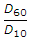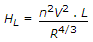# Civil Engineering - Water Supply Engineering

### Exercise :: Water Supply Engineering - Section 5

46.

The U.C. (uniformity coefficient)for the best filter media sand should be

 A. 2 B. 3 C. 4 D. 5 E. none of these.

Explanation:

No answer description available for this question. Let us discuss.

47.

Pick up the correct statement from the following :

 A. Deposition of calcium carbonate on the inside of the well pipe, causes incrustation of the pipe B. Incrustation of the pipe reduces the discharge C. Acidic waters cause corrosion of the pipes D. Corrosion results due to excessive withdrawal of sand along with water E. All the above.

Explanation:

No answer description available for this question. Let us discuss.

48.

Specific yield of a well is

 A. quantity of water than can be drawn from the well B. flow of water per unit time C. total quantity of water available in the well D. quantity of water per unit time per unit draw-down.

Explanation:

No answer description available for this question. Let us discuss.

49.

Normal values of overflow rate for plain sedimentation tanks in litres/hr/m2, generally range between

 A. 100 to 250 B. 250 to 500 C. 500 to 750 D. 750 to 1000

Explanation:

No answer description available for this question. Let us discuss.

50.

The formulafor the head loss in conduits is generally known as

 A. Hazen-William's formula B. Manning's formula C. Darcy-Weisbach formula D. Nikuradse formula.